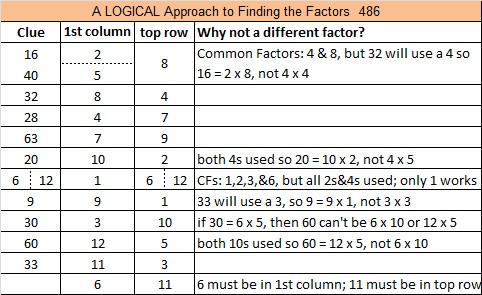# Finding √486 and Level 6

To find √486, I like to first see if 486 can be evenly divided by square numbers 4 or 9 because about 82% of numbers that can have their square roots reduced are divisible by 4 and/or by 9. It is also very easy to tell if a number can be evenly divided by 4 or by 9.

86 is not divisible by 4, so 486 is not divisible by 4.

However, 4 + 8 + 6 = 18, a multiple of 9 so 486 is divisible by 9, and now we know for sure that its square root can be reduced. 486 ÷ 9 = 54, another multiple of 9 so we get this two layer cake when we divide by 9 twice.Now take the square root of everything on the outside of the cake, and we get √486 = (√9)(√9)(√6) = 9√6.

Here is today’s Find the Factors puzzle:Print the puzzles or type the solution on this excel file: 12 Factors 2015-05-04

—————————————————————————————————

• 486 is a composite number.
• Prime factorization: 486 = 2 x 3 x 3 x 3 x 3 x 3, which can be written 486 = 2 x (3^5)
• The exponents in the prime factorization are 1 and 5. Adding one to each and multiplying we get (1 + 1)(5 + 1) = 2 x 6 = 12. Therefore 486 has exactly 12 factors.
• Factors of 486: 1, 2, 3, 6, 9, 18, 27, 54, 81, 162, 243, 486
• Factor pairs: 486 = 1 x 486, 2 x 243, 3 x 162, 6 x 81, 9 x 54, or 18 x 27
• Taking the factor pair with the largest square number factor, we get √486 = (√81)(√6) = 9√6 ≈ 22.04540—————————————————————————————————1.Paula Beardell Krieg
•ivasallay